Multimodal biometric system uses multiple biometric indicators (matchers) to identify the individuals.

In serial/sequential architecture, the outcome of one matcher may affect processing of subsequent sources as the acquisition and processing of sources takes place sequentially. In parallel architecture, the sources are processed independently and their outputs are combined. Both sequential and parallel architectures are quite commonly used but parallel is the advantageous and cost-effective one, as it is easier to acquire physically related biometric traits simultaneously. In case of acquiring multiple instances of the same trait, sequential architecture is preferred.

## Combination strategy:

This is a technique that is used to combine the outputs of individual classifiers.

## At the abstract level:

AND/OR rules

This is one of the simplest methods of combining decision outputs by different matchers. “AND” rules that if all the biometric matches agree that the input sample matches with the template, it is a match. “OR” rules that if at least one matcher decides that the input sample matches the output. With “AND” the False Accept Rate (FAR) is low and the False Reject Rate (FRR) is very high. While with “OR”, FAR is higher and FRR is lower. The combination of these two matchers amplifies errors and reduces the overall performance. So, the “AND” and “OR” rules are used rarely.

Majority voting

This is a common approach in which the sample is assigned to an identity on which a majority of the matchers agree. For R biometric matchers, at least k of the matchers agree on that identity. where,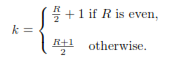When none of the identities are supported by k matchers i.e., when there are zero k matchers, a reject decision is given as output by the system.

Weighted majority voting

When the recognition accuracy of the of matchers used in a biometric system are not similar, assigning higher weights to the decisions made by more accurate matchers can be done.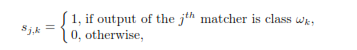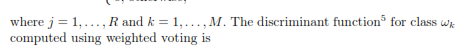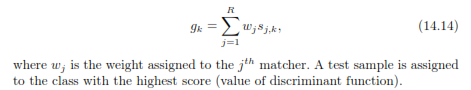## At the rank level

Highest rank method

In this method, each user is assigned the highest rank (r) as computed by different matchers.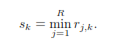This method is effective only when the number of users are more than the number of matchers. The strength of each of the matcher is utilised effectively.

Borda count method

This method uses the sum of ranks assigned by individual matchers to calculate the value of s.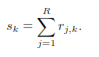Logistic regression method

This is a generalization of the Borda count method. The logistic regression method is useful when diﬀerent biometric matchers have signiﬁcant diﬀerences in their accuracies. However, this method requires a training phase to determine the weights.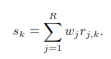## At the confidence level

The confidence level includes sum, mean, median, product, maximum and minimum rules. Difference score refers to the degree of change between two or more testings. Similarity score is the value of how much the data samples are alike.

The first step is to map the scores in common space and apply normalization to them. And fusion of the scores is done. An example is the handgeometry (difference score) and the fingerprints (similarity score), which give the difference score.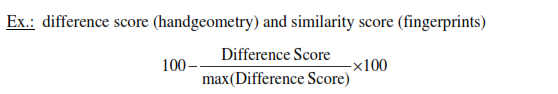Thus this explains the various algorithms involved in the functioning of the multimodal biometric systems.